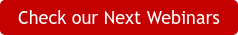You’ve probably learned how to make your own functions from our applied labs or from other resources, and know how to use them, but Python has included the most common ones for use in any program. The way you call them is the same was you call your own functions, but without having to define them first. We're going to take a look at the most important ones now, but be sure to look at the Python documentation for other useful functions that are available for use.

# str()

One of the most useful functions in Python is the string conversion function. Many times in scripting when you have a variable that contains an integer, or some other type of data, you may need to convert that value to a string, to print it out onto the screen.

In this example we have an integer in a variable, and we want to print it out, with the message. ‘The number is ‘ and the integer.We’re going to get an error, because what you’re asking Python to do is to add a integer and a string together, which doesn’t make sense. What needs to be done is to convert the number 10 into the string ‘10’. Python will understand that what we’re trying to do is not find a sum, but instead concatenate the two strings.

This is where the str function comes into play. It takes a value, and returns a string to represent it. In this case, you pass it a number, it will simply return the number, but in string format.Now that we’ve enclosed the number in str(), the code runs perfectly. The str function doesn’t have to take a number though – it can also take other data types, such as a boolean.# len()

Another common task you do on strings is to be able to find the length of them, and Python has a built in function for this. Let’s take the string ‘Hello World’ and try to find its length. We’re going to use the built-in len() function. We just need to pass the string we want to use, and it will return the number of characters in it:The len function can also be used to find the count of items in an object. You give it a string it’ll give you the number of characters, but you can also give it a list or tuple, and it will give you the number items in that particular object.

# int()

The built-in function to convert to an integer is called int(). The function will return the integer conversion of that number. This function does not round the input to the nearest integer, it simply disregards anything after the decimal point, and returns the number. So the input of 3.85 will return 3, not 4.Besides converting floats to integers, the int() can convert a string integer into an int data type.However a string that’s float can’t be converted into an integer using the int().# range()

The range function creates a list of numbers following a certain pattern. For example, you need a list containing all of the numbers 0 to 10. Instead of typing them out manually, you can use the range() to do it in one line.

Range accepts an integer as the parameter, in this case for our example, we’ll pass it 5. The function then starts at 0, and makes a list counting upwards until it hits one less than the number you put in. So, since the input is 5 , it will store the numbers 0 – 4 in the list of numbers.What if we wanted to print out a list of numbers from 5 - 10? We can include another argument for the starting number, so this will print the numbers 5 - 10.Now, can the range function print only the odd numbers under 12? Yes. The range function accepts a third value, which is the amount to go up each step. So if we start at 1, end at 12 and in steps of 2, we should end up with all the odd numbers under 12.These are the 4 most common built-in functions that you will encounter in your programming career. Now you can explore the Python documentation for more functions to play with. May the force be with you.Jaime Gabriel Jingco

Software Engineer/ Applied Labs Assistant Instructor### Min Yen